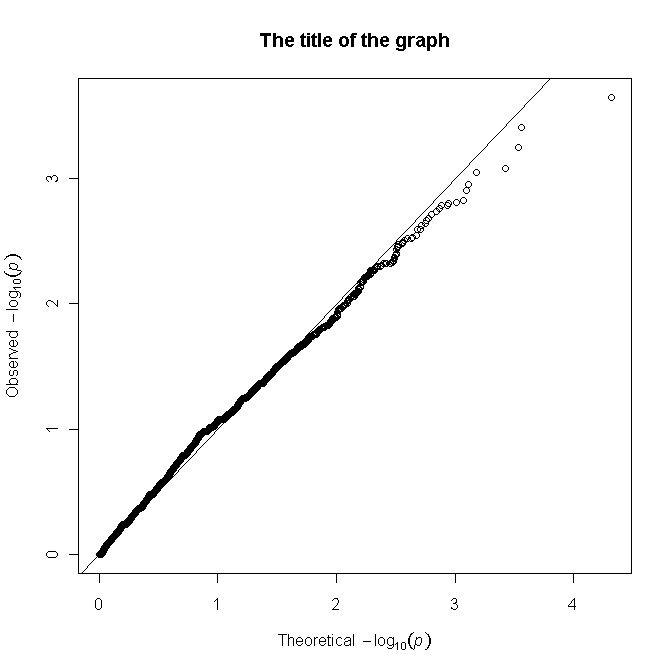Thursday, November 24, 2011

How to create qqplot in R

These are the simple instructions for creating a QQ-plot in R.

If you obtained a set of p-values from your experiment and want to check if they are different than the uniform distribution of p-values the QQ-plot is the right way to do this.
Save the file as file.txt with each p-value in a separate line.

log_obs_pvalues= -log10(obs_pvalues)
log_obs_pvalues <- c(log_obs_pvalues[,1])

If the values are not sorted:

log_obs_pvalues = sort(log_obs_pvalues)

Create uniform distribution of p-values using runif, where N is the number of observed p-values you have in file.txt:
uni_pvalues=runif(N)
log_uni_pvalues= -log10(uni_pvalues)
log_uni_pvalues = sort(log_uni_pvalues)

Simply do a scatter-plot of the the two variables:

plot (log_uni_pvalues,log_obs_pvalues, xlab=expression(Theoretical~~-log(italic(p))), ylab= expression(Observed~~-log(italic(p))), main="Title of your QQ-plot")

To draw the 45 degree line:
abline(0,1)

The resulting graph:From the following graph we can conclude that experimental p-values are not enriched with lower values than what would be expected according to the uniform distribution of p-values. For example, for the p-value of 0.05 the value -log10 equals ~1.3, so if the distribution of experimental p-values is enriched in significant values, the dots on the graph should go above the 45 degree line for Observed -log10(p)> 1.3.